# Common core subtraction - Number line subtraction

Again common core subtraction is using addition instead. Add up from the smaller number to the bigger number.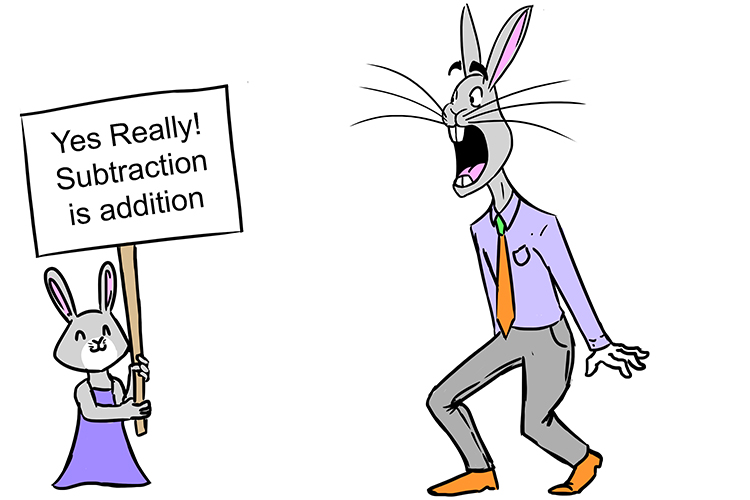Yes Really! Subtraction is addition.

Example 1

32-12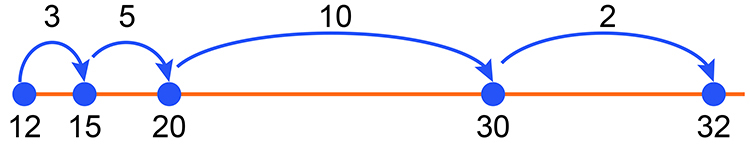3 + 5 + 10 + 2 20

To see this step by step

## Common core subtraction - Number line subtraction step by step

Example 1

32-12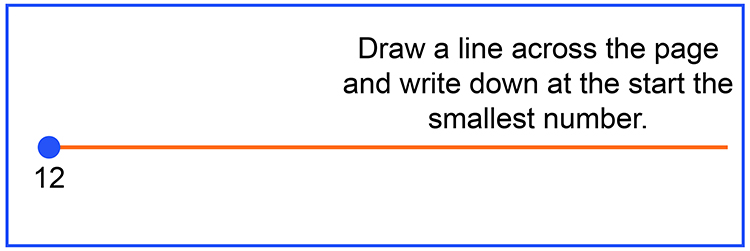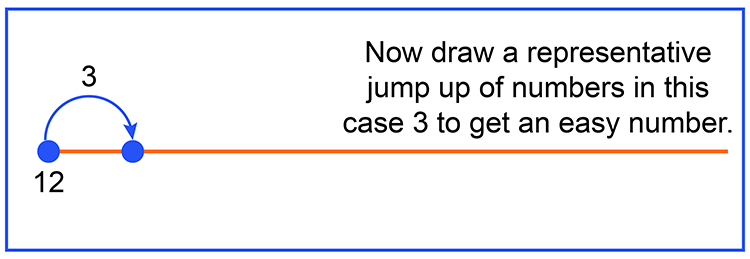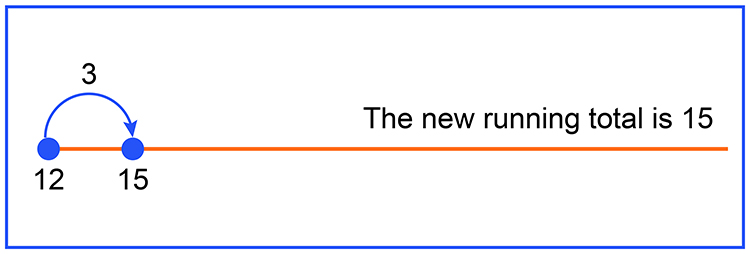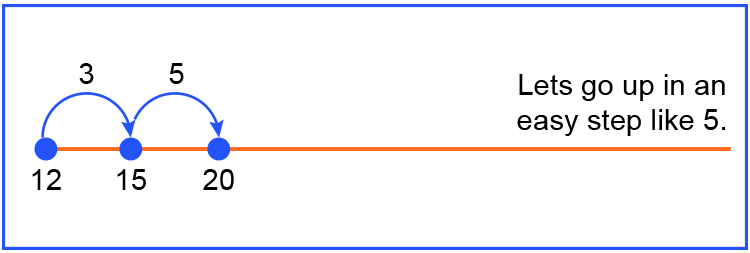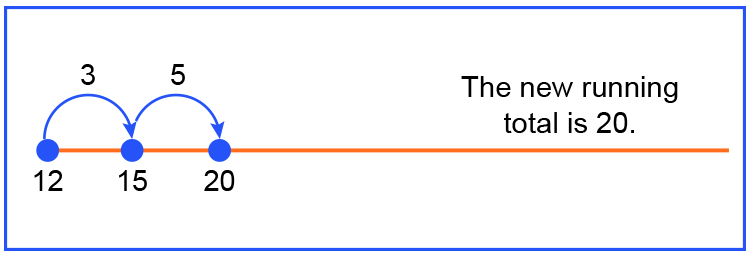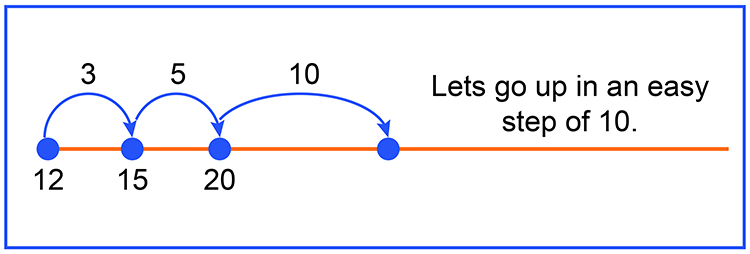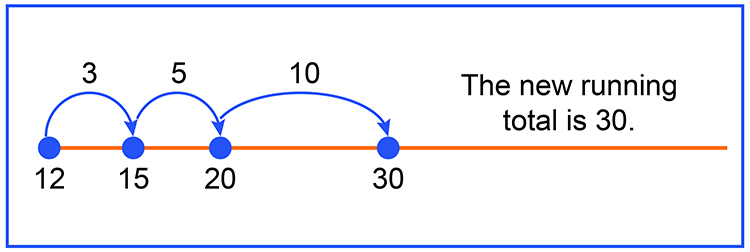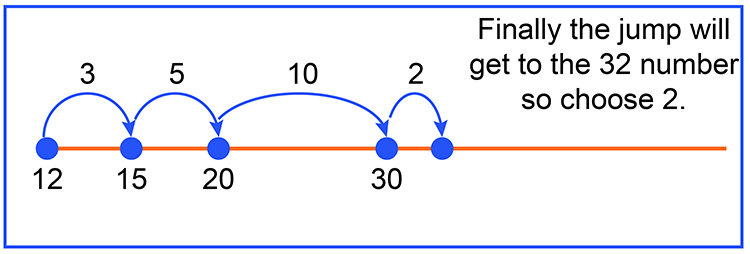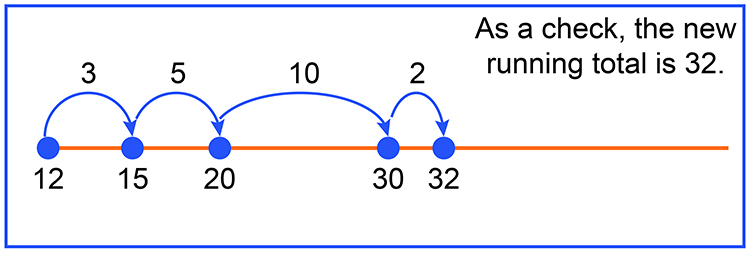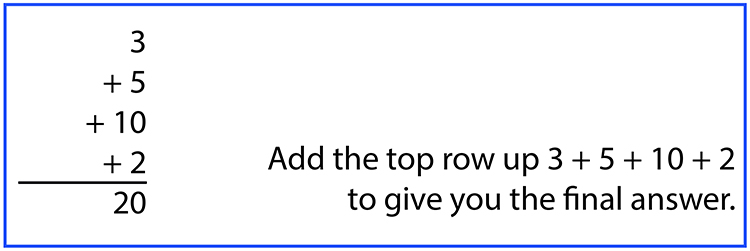Answer: 32-12=20

Answer: 32-12=20

Example 2

243-87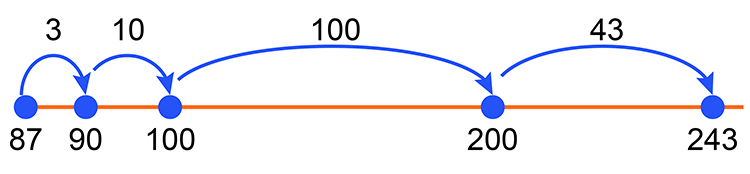3 + 10 + 100 + 43 156

To see this step by step

## Common core subtraction - Number line subtraction step by step

Example 2

243-87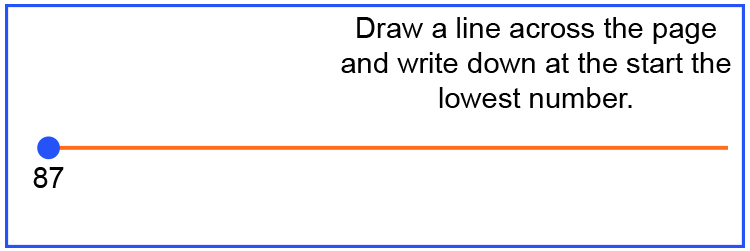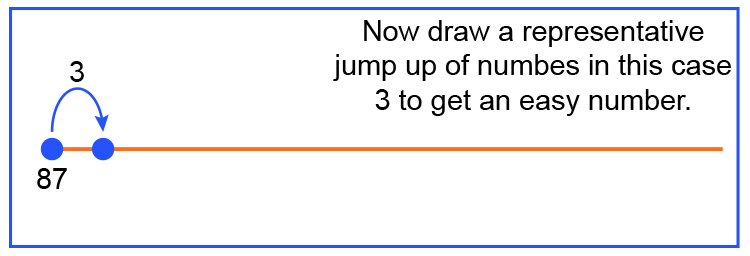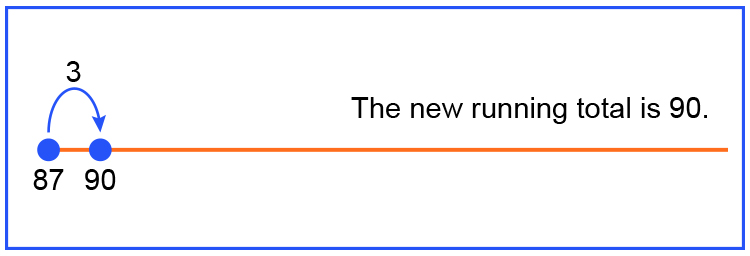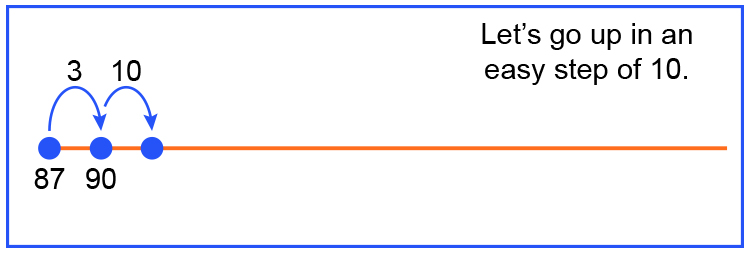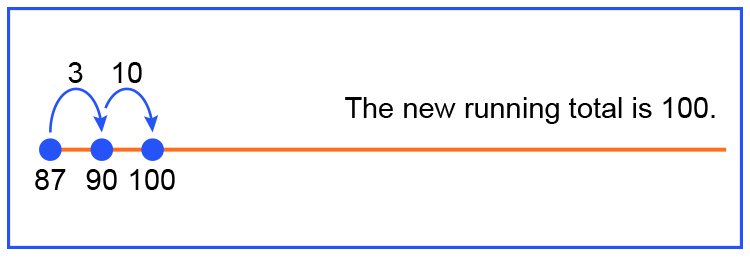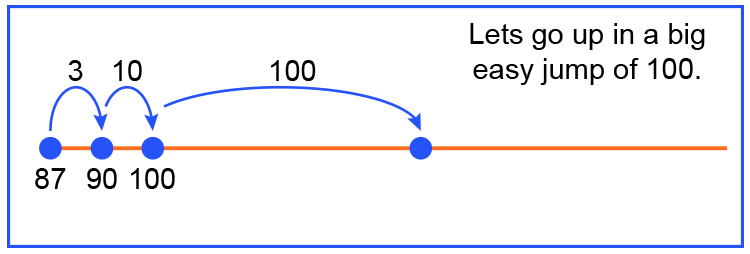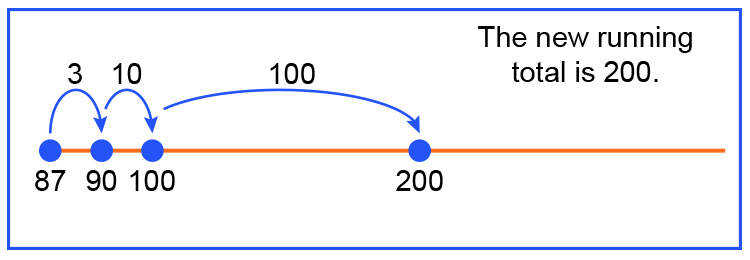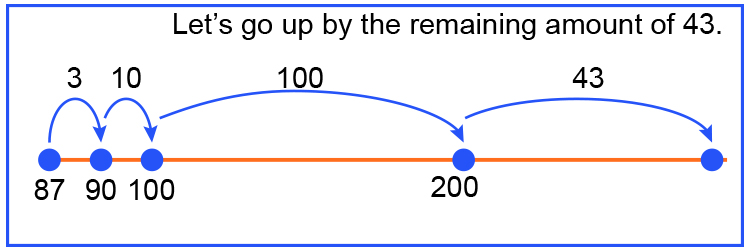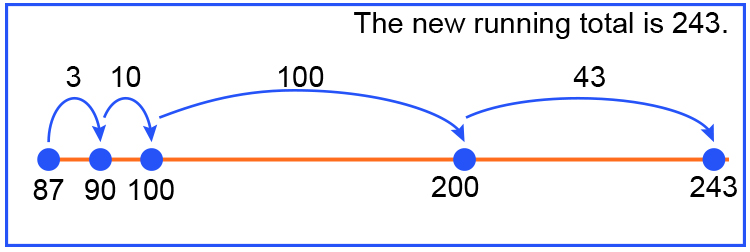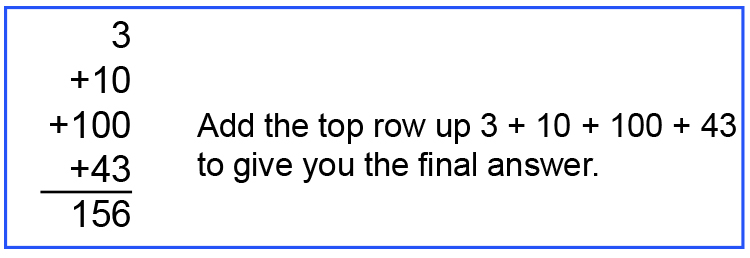Answer: 243-87=156

Answer: 243-87=156

Example 3

264-128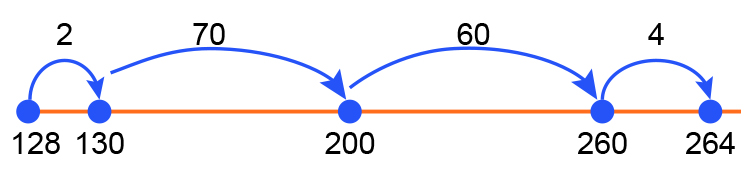2 + 70 + 60 + 4 136

To see this step by step

## Common core subtraction - Number line subtraction step by step

Example 3

264-128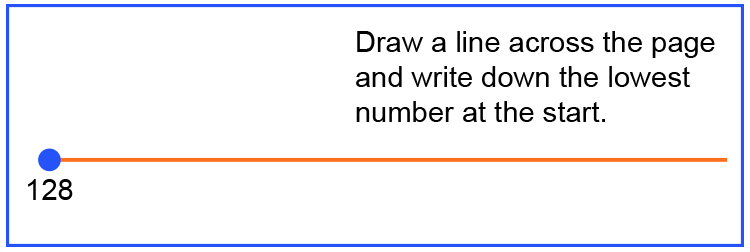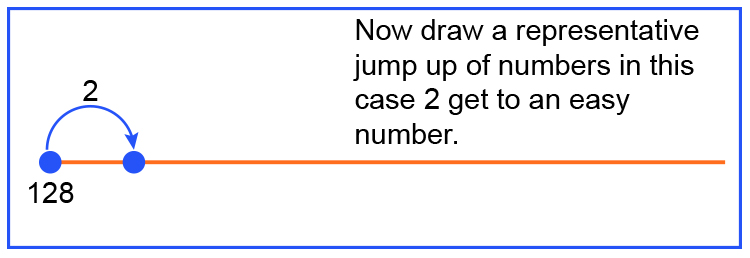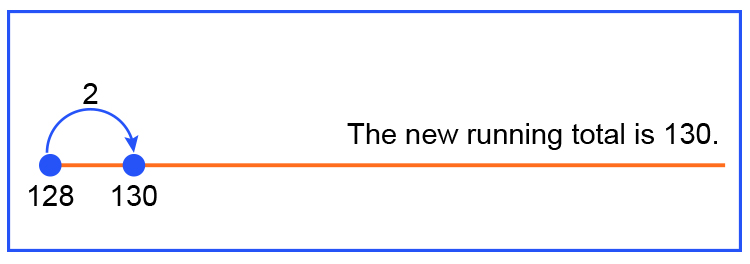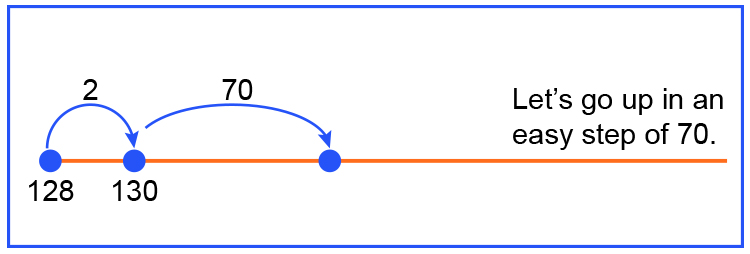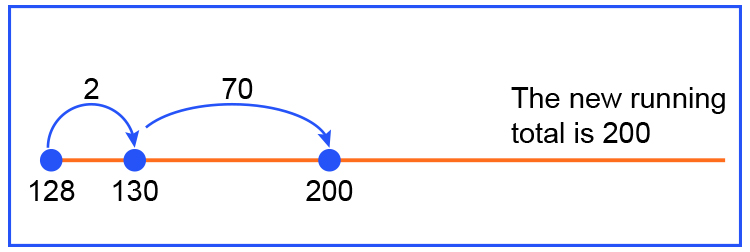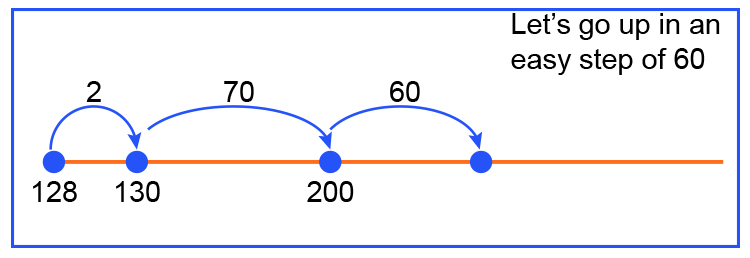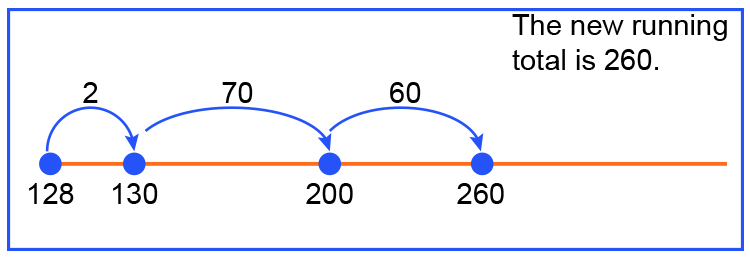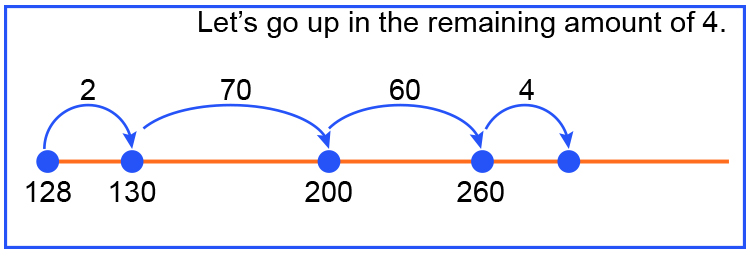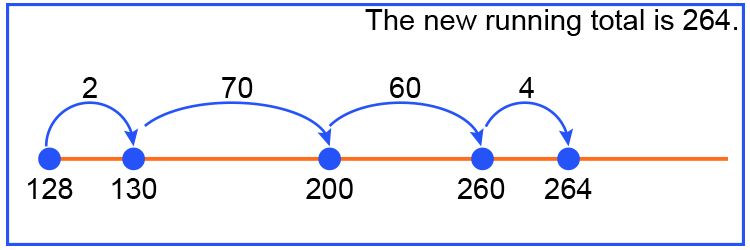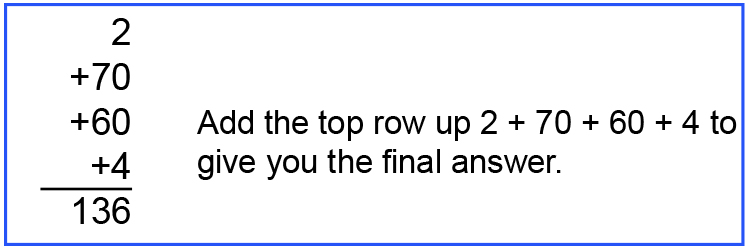Answer: 264-128=136

Answer: 264-128=136

OR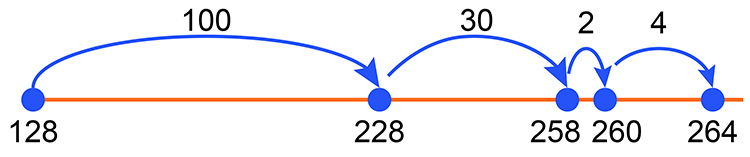100 + 30 + 2 + 4 136

To see this step by step

## Common core subtraction - Number line subtraction step by step

Example 3

264-128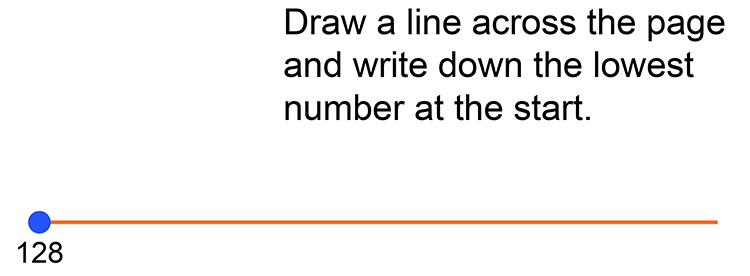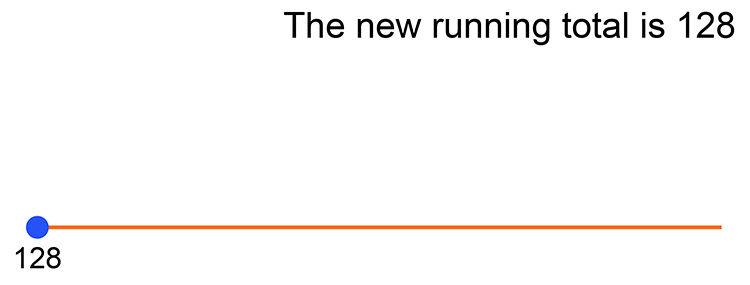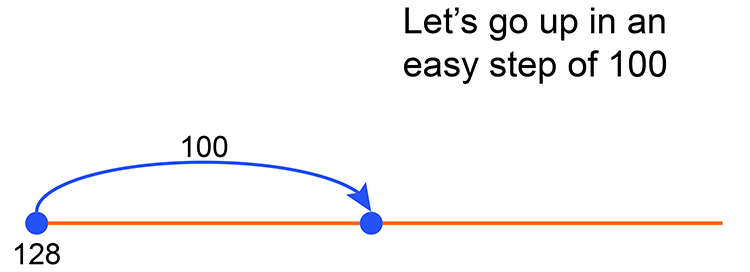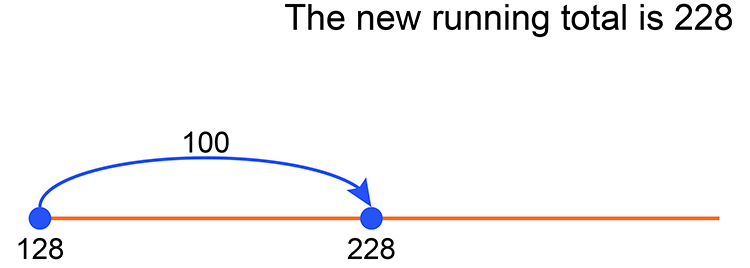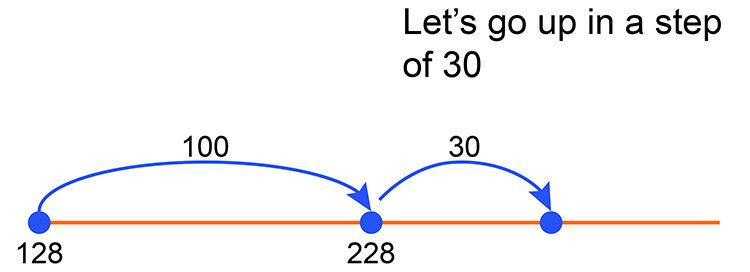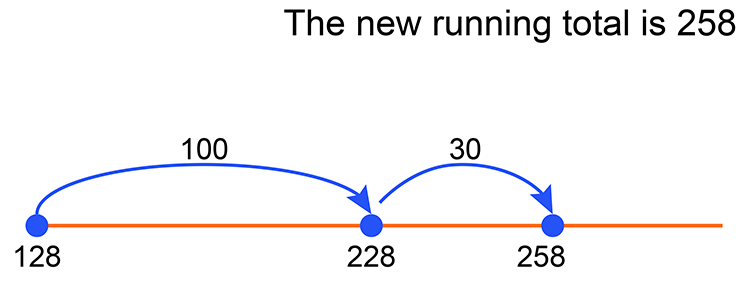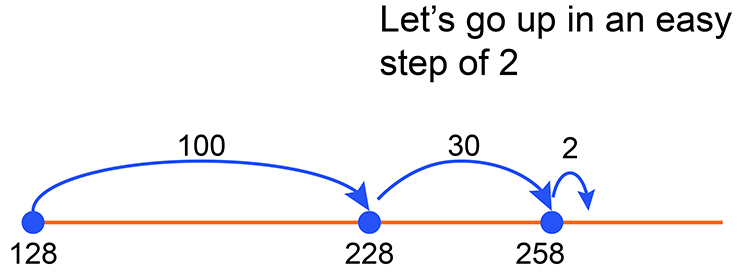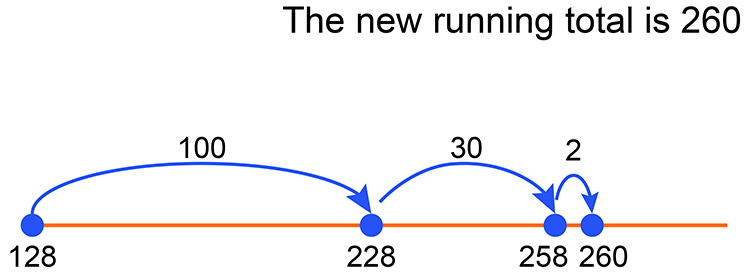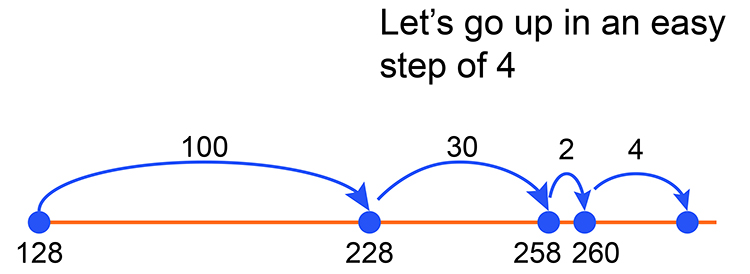Answer: 264-128=136